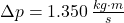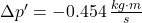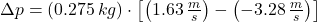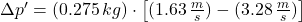## A ball with a mass of 275 g is dropped from rest, hits the floor, and re-bounds upward. If the ball hits the floor with a speed of 3.28 m/s,

Question

A ball with a mass of 275 g is dropped from rest, hits the floor, and re-bounds upward. If the ball hits the floor with a speed of 3.28 m/s, re-bounds with a speed of 1.63 m/s, and is in contact with the floor for 2.80 ms, determine the following.
A. Magnitude of the change in the ball’s momentum.
B. Change in the magnitude of the ball’s momentum.
C. Which of the two quantities calculated in parts (a) and (b) is more directly related to the net force acting on the ball during its collision with the floor?
A. Neither are related to the net force acting on the ball.
B. They both are equally related to the net force acting on the ball.
C. The change in the magnitude of the ball’s momentum.
D. The magnitude of the change in the ball’s momentum

in progress 0
2 months 2021-07-22T13:40:42+00:00 1 Answers 3 views 0

a), b), c) D. The magnitud of the change in the ball’s momentum.

Explanation:

a) The magnitude of the change in the ball’s momentum is:b) The change in the magnitude of the ball’s momentum:c) The magnitude of the change in the ball’s momentum is more directly related to the net force acting on the ball, as it measures the effect of the force on change in ball’s motion at measured time according to the Impact Theorem. So, the right answer is option D.